Journal of Global Change Data & Discovery2020.4(1):24-30

Citation：Tang, L., Ma, J. W., Shao, Z. Y., et al.Global Land Slope Frequency Dataset[J]. Journal of Global Change Data & Discovery,2020.4(1):24-30 .DOI: 10.3974/geodp.2020.01.04 .

DOI: 10

# Global Land Slope Frequency Dataset

## Tang, L.1  Ma, J. W.1  Shao, Z. Y.1  Peng, Q. Z.1,2*

1. Faculty of Land Resources Engineering, Kunming University of Science and Technology, Kunming 650093, China;

2. Surveying and Mapping Geo-Informatics Technology Research Center on Plateau Mountains of Yunnan Higher Education, Kunming 650093, China

Abstract: Slope frequency distribution can quantitatively measure slope, which is an important factor in landform characterization. The absence of high-resolution global slope frequency distribution hinders cross-regional comparative analysis. Obtaining 30 m digital elevation models (DEMs) covering a large land area—ASTER GDEM v3.0—permits quantitative slope analysis of Earth’s surface at an unprecedented scale and resolution. We used the ArcGIS Slope and Int routine to obtain the integer slope data in 90 intervals and then calculated the slope frequency distribution for 3 statistic units: 1° (latitude) ×1° (longitude) grids, the 7 continents, and the globe. These areas are different in scale, climate, and tectonic history, but their slope distributions are consistently unimodal. The peak in each distribution appears before 5°. 50% of the total ground surface has a slope less than 5.5°, the land surface slope of Oceania is the most gentle (μ = 5.23°) with the most concentrated distribution (σ = 5.31°), and the ice sheet surface slope of Antarctica is the steepest (μ = 13.53°) with the most dispersed distribution (σ = 15.86°). The data include the land slope frequency distributions for 3 statistic units, 1° (latitude) ×1° (longitude) grid with 22,205 data records in total, 7 continents, and the Earth in .xlsx format and the land slope frequency spatial distribution for the 1°x1° grids in .shp format. The dataset is archived in 631 data files, with a size of 232 MB (compressed to 54.5 MB in one file).

Keywords: globe; land; slope frequency distribution; ASTER GDEM grid

### 1 Introduction

The slope frequency distribution is the proportion of the total surface falling within certain slope classes, into which the total angular range of slope is subdivided. The slope frequency distribution is a powerful tool for describing topography and has been successfully employed for analysis of planetary landforms[2‒5], geologic hazards[6‒7], and regional landscapes and geomorphology[8‒9]. A slope frequency distribution can provide regional landform characteristics (e.g. the dominant slope angle) but is not easily comparable across regions. Hence, early studies focused on the identification of transformations of slope data to normalized slope distributions[10‒11]. Subsequent studies attempted to relate slope frequency distributions to landscape patterns[12–14]; however, regional slope analysis can only provide a reference for a single region because regional slope-frequency distributions show vastly different characteristics. Thus, it is necessary to establish a global benchmark for slope frequency distributions so that different studies can be compared.

The slope is a scale-dependent parameter that changes with the digital elevation model (DEM) resolution. As such slope is not comparable between DEMs with different resolutions. The first global land slope frequency distribution appeared in 1985 with a spatial resolution of 1° and has been used to compare the global topographic characteristics of Venus and Earth. Subsequently, the prevalent resolution of global DEM data, which is easy to obtain, has been refined to 1 (about 30 m); however, no study has calculated a global slope distribution using 30 m DEM data. In this contribution, the ASTER GDEM v3.0 DEM which nearly covers Earth’s entire land surface at 30 m resolution, was used to calculate the slope angle and generate a global land slope histogram, which can provide a reference for cross-regional slope analysis.

### 2 Metadata of the Dataset

The metadata of the “Global land slope frequency dataset” is summarized in Table 1. It includes the dataset full name, short name, authors, spatial resolution, data format, data size, data files, data publisher, and data sharing policy, etc.

Table 1  Metadata summary of “Global land slope frequency dataset”

 Items Description Dataset full name Global land slope frequency dataset Dataset short name LSF_Globe Authors Tang, L. D-4700-2019, Faculty of Land Resources Engineering, Kunming University of Science and Technology, 799643248@qq.com Ma, J. W. AAG-3726-2019, Faculty of Land Resources Engineering, Kunming University of Science and Technology, 359424547@qq.com Shao, Z. Y. AAG-3633-2019, Faculty of Land Resources Engineering, Kunming University of Science and Technology, 785383110@qq.com Peng, Q. Z. AAG-3629-2019, Faculty of Land Resources Engineering, Kunming University of Science and Technology, pqz20002@163.com Geographical region The land surface from 83°N to 83°S Data format .xlsx, .shp      Data size 54.5 MB (after compression) Data files 1°(latitude)´1°(longitude) grid land slope frequency distribution, 7 continents, and global land slope frequency distributions Foundation National Natural Science Foundation of China (41961039) Computing environment ArcGIS 10.2 (shared within the departmental research group) Data publisher Global Change Research Data Publishing & Repository, http://www.geodoi.ac.cn Address No. 11A, Datun Road, Chaoyang District, Beijing 100101, China Data sharing policy Data from the Global Change Research Data Publishing & Repository includes metadata, datasets (data products), and publications (in this case, in the Journal of Global Change Data & Discovery). Data sharing policy include: (1) Data are openly available and can be freely downloaded via the Internet; (2) End users are encouraged to use Data subject to citation; (3) Users, who are by definition also value-added service providers, are welcome to redistribute Data subject to written permission from the GCdataPR Editorial Office and the issuance of a Data redistribution license; and (4) If Data are used to compile new datasets, the ‘ten percent principal’ should be followed such that Data records utilized should not surpass 10% of the new dataset contents, while sources should be noted in suitable places in the new dataset Communication and searchable system DOI, DCI, CSCD, WDS/ISC, GEOSS, China GEOSS, Crossref

### 3 Methods

Slope data for slope frequency distribution analysis were computed using the 30 m ASTER GDEM v3.0 (GDEM v3), which provides land surface coverage of Earth from 83°N to 83°S latitude with 22,912 tiles. The elevation value of the ocean is 0 m which will influence the frequency value at 0° slope; hence, we use the 1:1,000,000 global base map data as a mask to remove the oceans from the GDEM v3. The global land slope frequency distribution dataset can be divided into three categories: 1° (latitude) × 1° (longitude) grid land slope frequency, 7 continents land slope frequency, and global land slope frequency.

The acquisition of slope frequency distribution data primarily required two steps: slope calculation and slope frequency calculation. Because the DEM dataset has 22,912 scenes in total, the scene by scene calculation takes a long time and is prone to errors; hence, a Python (v2.7.0) script was compiled to automate the calculation process.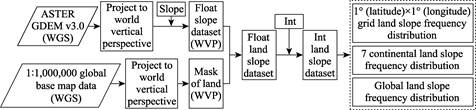Figure 1  Flowchart of the dataset processing

The projected coordinate system used in this study is the world vertical perspective (WVP), which has a vertical near-side perspective. The view height of WVP is 35800 km above the surface, just like viewing from a geosynchronous satellite. Because of minimal distortion near the center and maximum distortion near the edge, during projection, we take the center of each 1° × 1° grid as the observation center to ensure the smallest distortion of each tile (i.e., distortion is controlled within the range of one pixel). To obtain land slope frequency distributions, we first reprojected the DEM and 1:1,000,000 global base map data from WGS 1984 to WVP, and then used the ArcGIS Slope and Int routine to obtain the integer slope data. Finally, we used the 1:1,000,000 global base maps as a mask to remove the oceans from the slope dataset and calculated the land ratio for each 1° × 1° grid.

### 4 Results and Validation

4.1 Data Compostion

This global dataset was processed on a PC for about 120 hours, which is equipped with a single-core 2.66 GHz four core 8 thread CPU, 16 GB memory, and 5 independent hard disks for parallel reading and writing. The results of this data include one Excel file with 2 sheets and 90 vector files. All shapefiles are provided in the WGS84 geographic coordinate system and showed the 1° × 1° grid land slope frequency spatial distribution in .shp format. An excel file was created with land slope frequency distributions using 3 statistic units: 1° × 1° grid, each continent, and the globe. 707 DEM tiles contain little or no data after the oceans were removed; hence, we excluded these tiles from the dataset. The 1° × 1° grid land slope frequency data contain 22,205 records rather than 22,912 records, which is the total number of DEM tiles. In this paper the slope angle interval is 1°, hence the slope value range of [0°, 90°) is divided into 90 sections (i.e. each slope frequency data contains 90 frequency values). In the Excel file, the median slope value of each class is used to represent the individual slope class, that is, (i+0.5)° is used to represent the i-th slope section, and the range of this section is [i°, (i+1)°), i = 0, 1, 2, … 89.

4.2 Results

We chose 8  grids (1° × 1°) located in various typical relief regions, such as the Tibet Plateau, Kazak hills, Alps, Rockies, Amazon plain, Sahara desert, central plain of Oceania, and Antarctic glaciers, which respectively correspond to the figure numbers N33E086, N47E066, N47E012, N53W118, S03W066, N13E003, S30E141, and S77E014 (Figure 2). The slope frequency distribution of each grid is similar to that of the continent where it is located. The change in frequency-slope trend firstly increases and then decreases, except for the Alps and Antarctic glaciers. Results show that the land slope frequency distribution for different landforms may be similar, and the shape of the frequency curve is mostly unimodal with a long tail and right skewness.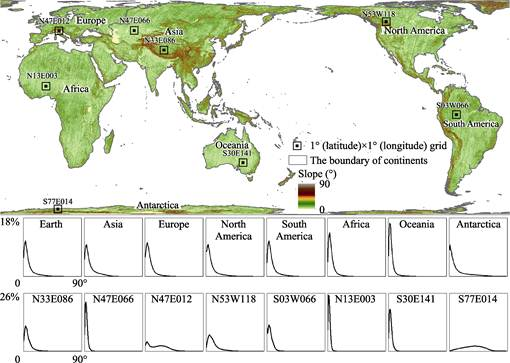Figure 2  Global land slope map, and slope frequency distributions in each continent, the Earth, and typical geomorphic regions, which noted as 1° (latitude) ×1° (longitude) grid

The land slope distributions of the 22,205 1° by 1° grids are summarized in Figure 3, where the 1st percentile was used to replace the minimum value, and the 99th percentile was used to replace the maximum value, to avoid the influence of extreme values. The frequency value corresponding to the 99th percentile of 0.5° is 74.26%. From the box chart (Figure 3) and the land slope frequency distributions of each continent and the entire globe (Figure 2), we find the frequency values are all increasing to the maximum before 5°, and then rapidly decrease with increasing slope after the peak. 50% of the total land surface has a slope of less than 5.5° (Figures 2 and 4). The ground in Oceania is the flattest (μ = 5.23°) with the most concentrated distribution (σ = 5.31°). 76% of the Oceanian ground has a slope steeper than 6° (Figure 4, Table 2). The ice sheet in Antarctica has the steepest slope (μ = 13.53°) with the most scattered distribution (σ = 15.86°, Figure 4). 60% of the Antarctic land surface has a slope of less than 7° (Table 2).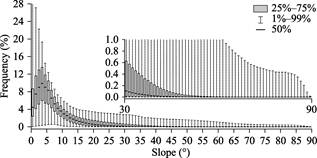Figure 3  Box chart of 22,205 DEM tiles slope frequency distribution

4.3 Data Validation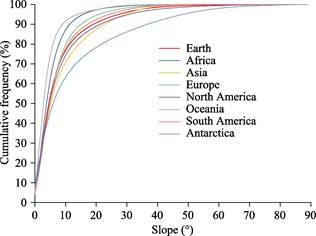Figure 4  Cumulative frequency distributions of slope in 7 continents and the total Earth land surface

The slope frequency distribution is a quantitative analytical tool for slope analysis. However, slope accuracy depends on the DEM data. The ASTER GDEM v3.0 data were created from the automated processing of the entire ASTER Level 1A archive of scenes acquired between March 1, 2000, and November 30, 2013. The ASTER GDEM Version 3 data products offer a substantial improvement over Version 2 products. Although some relief changes during the data acquisition period, on a global scale the impact of these local topographic changes can be ignored. Figure 2 shows that Antarctica and Greenland have a high ground surface slope. Because ice and snow-covered areas have high optical reflectivity, a stereo correlation was used to produce the ASTER DEM. Therefore, DEM data in this area have poor quality and so do the slope frequency distribution data. We suggest that the slope frequency distribution data for Antarctica and Greenland in this data set be avoided in subsequent research.

Table 2  Land slope frequency distribution for different statistic units

 Statistic unit Mean value μ (°) Standard deviation σ (°) Statistic unit Mean value μ (°) Standard deviation σ (°) Africa 6.25 5.38 South America 8.25 7.77 Asia 9.63 9.34 Oceania 5.23 5.31 Europe 7.70 6.98 Antarctica 13.53 15.86 North America 9.43 10.74 Earth 8.63 9.28

### 5 Discussion and Conclusion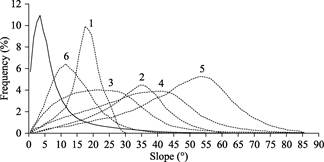Figure 5  Slope frequency distributions from previous studies (dash line) and a global land slope distribution (solid line) 1—Lucore hollow a mature basin; 2—the Northwestern Himalayas; 3—wash number is 4 mm·yr‒1 throughout the continental United States; 4—wash number is 8mm·yr‒1 throughout the continental United States; 5—wash number is 16 mm·yr‒1 throughout the continental United States; 6—landslide areas in Upper Tiber River basin

We suggest that slope frequency distributions vary between the study areas and the size and landforms of the study areas[1,8,12,21]. Slope distributions significantly vary (dash line, Figure 5); hence, we can neither compare across regions nor compare with statistical models. However, if compared with a global land slope frequency distribution (solid line, Figure 5), we can easily and quantitatively describe ground slope in a global uniform context. For example, the peak slope of curve 6 in Figure 5 is the closest to the global land peak slope, indicating that the terrain slope of the study area is relatively gentle. Similarly, the terrain slope of the study area represented by curve 5 is the most rugged.

The slope is a strong scale-dependent parameter that cannot be compared across different DEM resolutions. To date, few studies of global slope frequency distribution have been conducted at high resolution. As a useful parameter in earth sciences, which can provide quantitative characteristics for describing ground surfaces, the global slope frequency distribution needs to match the common 30 m DEM resolution. 30 m is one of the common free DEM resolutions. The slope frequency distribution generated from ASTER GDEM v3.0 can provide a global reference for slope frequency analysis (e.g. landslide, geomorphic). This dataset includes land slope frequency distributions for 3 statistical units: 1° (latitude) ´ 1° (longitude) grids, the 7 continents, and the entire globe, that enrich regional and global benchmarks. We hope this suite of land slope frequency distributions will facilitate future quantitative cross-region slope analysis at the 30 m resolution. Because slope frequency distribution data might vary for different DEMs generated from different data sources, this data set can only be used as a reference for studies based on GDEM v3.0 data.

### Author Contributions

Peng, Q. Z. designed the dataset processing. Tang, L. and Ma, J. W. designed the algorithms for the dataset. Ma, J. W. and Shao, Z. Y. contributed to data processing and analysis. Tang, L. wrote the data paper.

### References

    NASA, METI. ASTER GDEM v3.0 [Z]. https://lpdaac.usgs.gov/.

    Guzzetti, F., Ardizzone, F., Cardinali, M., et al. Distribution of landslides in the Upper Tiber River basin, central Italy [J]. Geomorphology, 2008, 96(1): 105-122.